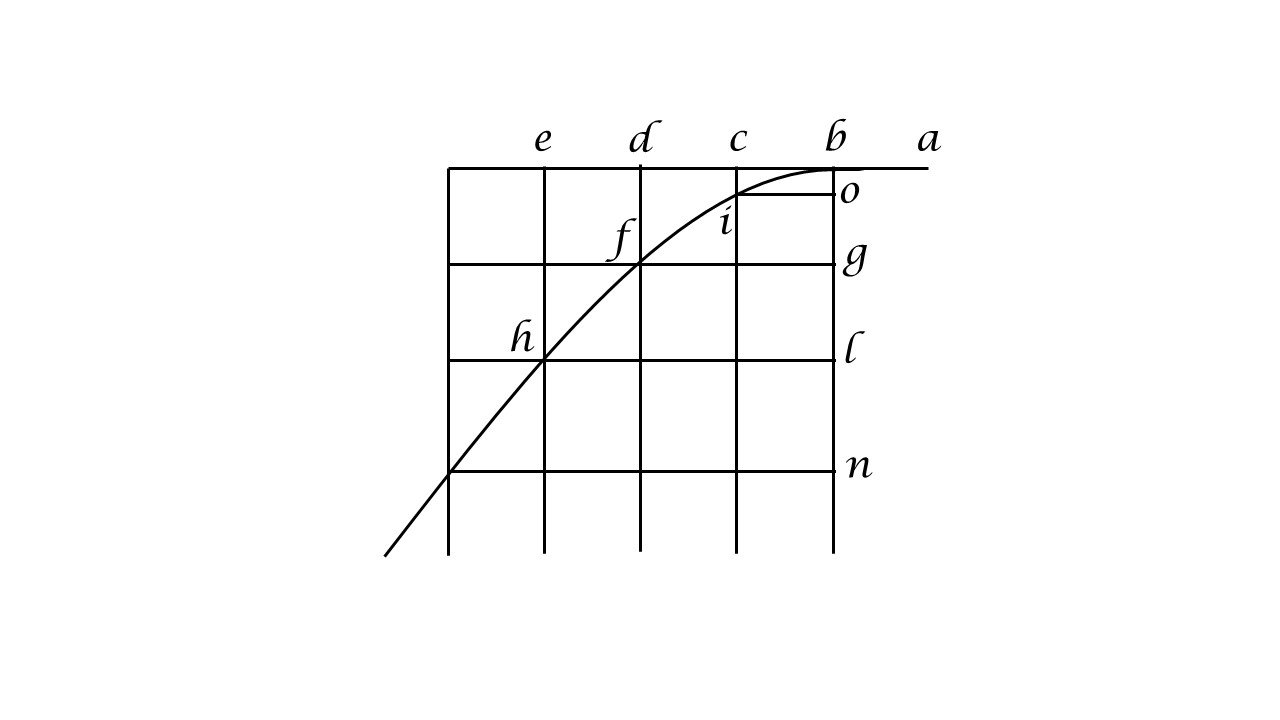iSoul In the beginning is reality.

# Interchange of length and duration

Length and duration are independent measures of the extent of motion, which are measured by comparing the target motion to a uniform reference motion. Although uniform linear motion is simpler in theory, uniform circular motion is simpler in practice – especially for unstopped motion. With one addition, the classic circular clock with hands serves as a reference motion. The addition is to mark the circumference in length units along with the duration units of the angles between the hands and the vertical. See post on a metreloge here.

Galileo uses horizontal uniform linear motion to mark length and duration below (from his Dialogues Concerning Two New Sciences, Fourth Day):The horizontal uniform motion of a particle coming from the right at a-b is continued with b-c-d-e as the horizontal component of the particle descending with uniform acceleration b-o-g-l-n. The vertical component represents the dependent variable, which has the form of a semi-parabola. The uniform horizontal motion, which could be any in direction, is the independent variable in units of either length or duration.

To interchange length and duration in an equation with a parametric function of time requires four steps: (1) with a change of variables switch the independent and dependent variables, time and stance; (2) linearize the stance, that is, break its dependent relation; (3) bring time under a functional relation with the new parameter, stance; and (4) expand time to include angular components. Functions are inverted and the independent and dependent status of variables is switched. An inversion and a kind of re-inversion return to the same function.

In the example above, the horizontal uniform motion which was taken by Galileo to represent time is re-conceived to represent the independent length variable, stance. The constant acceleration of the vertical component is re-conceived to represent the dependent duration variable with constant lenticity. The quadratic sequence in units of length becomes a sequence in units of duration at a constant rate.

The result of this interchange process is that the equations of motion for length and duration are interchangeable without functional change. All of the equations of physics in terms of parametric functions of time may be adopted as parametric functions of stance. In that sense it would be best to abstract a functional representation that applies to both length and duration, time and stance.